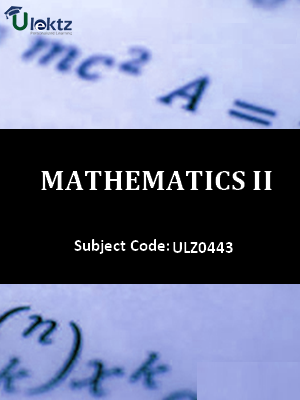uLektz apps

•My WalletMy Order
•My Profile
•My Connections
•My Books
•My Videos
•My Tests
•My Calender
•My Messages
•My Shopping Cart
•My Orders
•Account Settings
•Help

uLektz apps

# Book Details# Mathematics-II

Course Code:ULZ0443

Author:uLektz

University:

Regulation:2018

Categories:Electronics & Communication

Format :ePUB3 (DRM Protected)

Type :eBook

FREE

Description :Mathematics-II of ULZ0443 covers the latest syllabus prescribed by General for All University for regulation 2018. Author: uLektz, Published by uLektz Learning Solutions Private Limited.

Note : No printed book. Only ebook. Access eBook using uLektz apps for Android, iOS and Windows Desktop PC.

##### Topics
###### UNIT 1 MATRICES

1.1 Types of Matrices: Symmetric, Skew-symmetric and Orthogonal Matrices; Complex Matrices, Inverse and Rank of matrix using elementary transformations, Rank-Nullity theorem

1.2 System of linear equations, Characteristic equation

1.3 Cayley-Hamilton Theorem and its application

1.4 Eigen values and eigenvectors

1.5 Diagonalisation of a Matrix

###### UNIT 2 DIFFERENTIAL CALCULUS- I

2.1 Introduction to limits, continuity and differentiability, Continuity, Derivatives

2.2 Rolle’s Theorem, Lagrange’s Mean value theorem and Cauchy mean value theorem

2.3 Successive Differentiation (nth order derivatives), Leibnitz theorem and its application

2.4 Envelope, Involutes and Evolutes

2.5 Curve tracing: Cartesian and Polar co-ordinates.

###### UNIT 3 DIFFERENTIAL CALCULUS-II

3.1 Partial derivatives

3.2 Total derivative

3.3 Euler’s Theorem for homogeneous functions

3.4 Taylor and Maclaurin’s theorems for a function of one and two variables

3.5 Maxima and Minima of functions of several variables, Lagrange Method of Multipliers

3.6 Jacobians

3.7 Approximation of errors

###### UNIT 4 MULTIVARIABLE CALCULUS-I

4.1 Multiple integration: Double integral, Triple integral, Change of order of integration

4.2 Change of variables

4.3 Application: Areas and volumes, Center of mass and center of gravity (Constant and variable densities)

###### UNIT 5 VECTOR CALCULUS

5.1 Vector differentiation: Gradient, Curl and Divergence and their Physical interpretation, Directional derivatives, Tangent and Normal planes

5.2 Vector Integration: Line integral, Surface integral, Volume integral

5.3 Gauss’s Divergence theorem, Green’s theorem

5.4 Stoke’s theorem ( without proof) and their applications

### Related Books